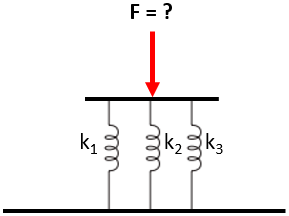## Springs in Parallel

Consider three springs in parallel have the following spring constants (from left to right): 4 N/m, 2 N/m, & 3 N/m. What force is required to compress the springs 5 m?Hint
The force in a spring is:
$$F_s=k\delta$$$where $$k$$ is the spring constant, and $$\delta$$ is the change in spring length from the un-deformed spring length. Hint 2 The spring constant for springs in parallel: $$k_{eq}=\sum_{i} k_i$$$
First, let’s determine the equivalent spring constant for several springs in parallel:
$$k_{eq}=\sum_{i} k_i$$$Since the problem statement has three springs in parallel: $$k_{eq}=k_1+k_2+k_3=4\frac{N}{m}+2\frac{N}{m}+3\frac{N}{m}=9\:N/m$$$
A spring’s deflection and force are related by:
$$F_s=k\delta$$$where $$F_s$$ is the forced applied to the spring, $$k$$ is the spring constant, and $$\delta$$ is the change in spring length from the un-deformed spring length. $$F_s=9\frac{N}{m}\times 5m=45\:N$$$
45 N